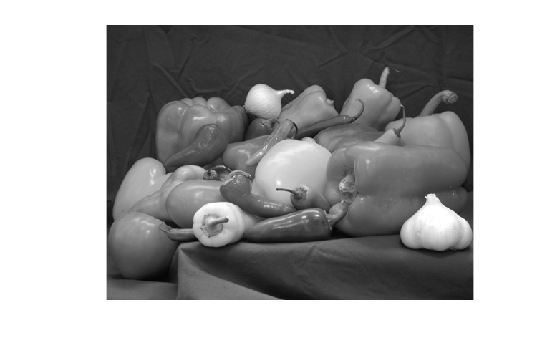# rgb2lab

Convert RGB to CIE 1976 L*a*b*

## Syntax

``lab = rgb2lab(RGB)``
``lab = rgb2lab(RGB,Name,Value)``

## Description

example

````lab = rgb2lab(RGB)` converts sRGB values to CIE 1976 L*a*b* values.```

example

````lab = rgb2lab(RGB,Name,Value)` specifies additional conversion options, such as the color space of the RGB image, using one or more name-value pair arguments.```

## Examples

collapse all

Use `rgb2lab` to convert the RGB white value to L*a*b.

`rgb2lab([1 1 1])`
```ans = 1×3 100.0000 0 0.0000 ```

Convert an Adobe RGB (1998) color value to L*a*b* using the `ColorSpace` parameter.

`rgb2lab([.2 .3 .4],'ColorSpace','adobe-rgb-1998')`
```ans = 1×3 30.1783 -5.6902 -20.8223 ```

Use `rgb2lab` to convert an RGB color to L*a*b using the D50 reference white.

`rgb2lab([.2 .3 .4],'WhitePoint','d50')`
```ans = 1×3 31.3294 -4.0732 -18.1750 ```

Read RGB image into the workspace.

`rgb = imread('peppers.png');`

Convert the RGB image to the L*a*b* color space.

`lab = rgb2lab(rgb);`

Display the L* component of the L*a*b* image.

`imshow(lab(:,:,1),[0 100])`## Input Arguments

collapse all

RGB color values to convert, specified as a numeric array in one of these formats.

• c-by-3 colormap. Each row specifies one RGB color value.

• m-by-n-by-3 image

• m-by-n-by-3-by-p stack of images

Data Types: `single` | `double` | `uint8` | `uint16`

### Name-Value Arguments

Specify optional pairs of arguments as `Name1=Value1,...,NameN=ValueN`, where `Name` is the argument name and `Value` is the corresponding value. Name-value arguments must appear after other arguments, but the order of the pairs does not matter.

Example: ```lab = rgb2lab([0.25 0.40 0.10],WhitePoint="d50")```

Before R2021a, use commas to separate each name and value, and enclose `Name` in quotes.

Example: `lab = rgb2lab([0.25 0.40 0.10],"WhitePoint","d50")`

Color space of the input RGB values, specified as `"srgb"`, `"adobe-rgb-1998"`, `"prophoto-rgb"`, or `"linear-rgb"`. If you specify `"linear-rgb"`, then `rgb2lab` assumes the input RGB values are linearized sRGB values.

Data Types: `string` | `char`

Reference white point, specified as a 1-by-3 vector or one of the CIE standard illuminants listed in the table.

ValueWhite Point
`"a"`

CIE standard illuminant A, `[1.0985, 1.0000, 0.3558]`. Simulates typical, domestic, tungsten-filament lighting with correlated color temperature of 2856 K.

`"c"`CIE standard illuminant C, `[0.9807, 1.0000, 1.1822]`. Simulates average or north sky daylight with correlated color temperature of 6774 K. Deprecated by CIE.
`"e"`Equal-energy radiator, `[1.000, 1.000, 1.000]`. Useful as a theoretical reference.
`"d50"`CIE standard illuminant D50, `[0.9642, 1.0000, 0.8251]`. Simulates warm daylight at sunrise or sunset with correlated color temperature of 5003 K. Also known as horizon light.

`"d55"`

CIE standard illuminant D55, `[0.9568, 1.0000, 0.9214]`. Simulates mid-morning or mid-afternoon daylight with correlated color temperature of 5500 K.

`"d65"`CIE standard illuminant D65, `[0.9504, 1.0000, 1.0888]`. Simulates noon daylight with correlated color temperature of 6504 K.
`"icc"`Profile Connection Space (PCS) illuminant used in ICC profiles. Approximation of `[0.9642, 1.000, 0.8249]` using fixed-point, signed, 32-bit numbers with 16 fractional bits. Actual value: ```[31595,32768, 27030]/32768```.

Data Types: `single` | `double` | `string` | `char`

## Output Arguments

collapse all

Converted L*a*b* color values, returned as a numeric array of the same size as the input. The output type is `double` unless the input type is `single`, in which case the output type is also `single`.

AttributeDescription
L*Luminance or brightness of the image. Values are in the range [0, 100], where 0 specifies black and 100 specifies white. As L* increases, colors become brighter.
a*Amount of red or green tones in the image. A large positive a* value corresponds to red/magenta. A large negative a* value corresponds to green. Although there is no single range for a*, values commonly fall in the range [-100, 100] or [-128, 127).
b*Amount of yellow or blue tones in the image. A large positive b* value corresponds to yellow. A large negative b* value corresponds to blue. Although there is no single range for b*, values commonly fall in the range [-100, 100] or [-128, 127).

Data Types: `double` | `single`

## Tips

• If you specify the input RGB color space as `"linear-rgb"`, then `rgb2lab` assumes the input values are linearized sRGB values. If instead you want the input color space to be linearized Adobe RGB (1998), then you can use the `lin2rgb` function.

For example, to convert linearized Adobe RGB (1998) image `RGBlinadobe` to the CIE 1976 L*a*b* color space, perform the conversion in two steps:

```RGBadobe = lin2rgb(RGBlinadobe,"ColorSpace","adobe-rgb-1998"); LAB = rgb2lab(RGBadobe,"ColorSpace","adobe-rgb-1998"); ```

## Version History

Introduced in R2014b

expand all